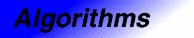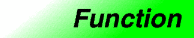# set_differenceCategory: algorithms Component type: function

## Prototype

`Set_difference` is an overloaded name; there are actually two `set_difference` functions.

```template <class InputIterator1, class InputIterator2, class OutputIterator>
OutputIterator set_difference(InputIterator1 first1, InputIterator1 last1,
InputIterator2 first2, InputIterator2 last2,
OutputIterator result);

template <class InputIterator1, class InputIterator2, class OutputIterator,
class StrictWeakOrdering>
OutputIterator set_difference(InputIterator1 first1, InputIterator1 last1,
InputIterator2 first2, InputIterator2 last2,
OutputIterator result,
StrictWeakOrdering comp);
```

## Description

`Set_difference` constructs a sorted range that is the set difference of the sorted ranges `[first1, last1)` and `[first2, last2)`. The return value is the end of the output range.

In the simplest case, `set_difference` performs the "difference" operation from set theory: the output range contains a copy of every element that is contained in `[first1, last1)` and not contained in `[first2, last2)`. The general case is more complicated, because the input ranges may contain duplicate elements. The generalization is that if a value appears `m` times in `[first1, last1)` and `n` times in `[first2, last2)` (where `m` or `n` may be zero), then it appears `max(m-n, 0)` times in the output range.  `Set_difference` is stable, meaning both that elements are copied from the first range rather than the second, and that the relative order of elements in the output range is the same as in the first input range.

The two versions of `set_difference` differ in how they define whether one element is less than another. The first version compares objects using `operator<`, and the second compares objects using a functors `comp`.

## Definition

Defined in the standard header algorithm, and in the nonstandard backward-compatibility header algo.h.

## Requirements on types

For the first version:

• `InputIterator1` is a model of InputIterator.
• `InputIterator2` is a model of InputIterator.
• `OutputIterator` is a model of OutputIterator.
• `InputIterator1` and `InputIterator2` have the same value type.
• `InputIterator`'s value type is a model of LessThanComparable.
• The ordering on objects of `InputIterator1`'s value type is a strict weak ordering, as defined in the LessThanComparable requirements.
• `InputIterator`'s value type is convertible to a type in `OutputIterator`'s set of value types.

For the second version:

• `InputIterator1` is a model of InputIterator.
• `InputIterator2` is a model of InputIterator.
• `OutputIterator` is a model of OutputIterator.
• `StrictWeakOrdering` is a model of StrictWeakOrdering.
• `InputIterator1` and `InputIterator2` have the same value type.
• `InputIterator1`'s value type is convertible to `StrictWeakOrdering`'s argument type.
• `InputIterator`'s value type is convertible to a type in `OutputIterator`'s set of value types.

## Preconditions

For the first version:

• `[first1, last1)` is a valid range.
• `[first2, last2)` is a valid range.
• `[first1, last1)` is ordered in ascending order according to `operator<`. That is, for every pair of iterators `i` and `j` in `[first1, last1)` such that `i` precedes `j`, `*j < *i` is `false`.
• `[first2, last2)` is ordered in ascending order according to `operator<`. That is, for every pair of iterators `i` and `j` in `[first2, last2)` such that `i` precedes `j`, `*j < *i` is `false`.
• There is enough space to hold all of the elements being copied. More formally, the requirement is that `[result, result + n)` is a valid range, where `n` is the number of elements in the difference of the two input ranges.
• `[first1, last1)` and `[result, result + n)` do not overlap.
• `[first2, last2)` and `[result, result + n)` do not overlap.

For the second version:

• `[first1, last1)` is a valid range.
• `[first2, last2)` is a valid range.
• `[first1, last1)` is ordered in ascending order according to `comp`. That is, for every pair of iterators `i` and `j` in `[first1, last1)` such that `i` precedes `j`, `comp(*j, *i)` is `false`.
• `[first2, last2)` is ordered in ascending order according to `comp`. That is, for every pair of iterators `i` and `j` in `[first2, last2)` such that `i` precedes `j`, `comp(*j, *i)` is `false`.
• There is enough space to hold all of the elements being copied. More formally, the requirement is that `[result, result + n)` is a valid range, where `n` is the number of elements in the difference of the two input ranges.
• `[first1, last1)` and `[result, result + n)` do not overlap.
• `[first2, last2)` and `[result, result + n)` do not overlap.

## Complexity

Linear. Zero comparisons if either `[first1, last1)` or `[first2, last2)` is empty, otherwise at most `2 * ((last1 - first1) + (last2 - first2)) - 1` comparisons.

## Example

```inline bool lt_nocase(char c1, char c2) { return tolower(c1) < tolower(c2); }

int main()
{
int A1[] = {1, 3, 5, 7, 9, 11};
int A2[] = {1, 1, 2, 3, 5, 8, 13};
char A3[] = {'a', 'b', 'b', 'B', 'B', 'f', 'g', 'h', 'H'};
char A4[] = {'A', 'B', 'B', 'C', 'D', 'F', 'F', 'H' };

const int N1 = sizeof(A1) / sizeof(int);
const int N2 = sizeof(A2) / sizeof(int);
const int N3 = sizeof(A3);
const int N4 = sizeof(A4);

cout << "Difference of A1 and A2: ";
set_difference(A1, A1 + N1, A2, A2 + N2,
ostream_iterator<int>(cout, " "));
cout << endl
<< "Difference of A3 and A4: ";
set_difference(A3, A3 + N3, A4, A4 + N4,
ostream_iterator<char>(cout, " "),
lt_nocase);
cout << endl;
}
```

The output is

```Difference of A1 and A2: 7 9 11
Difference of A3 and A4: B B g H
```